Courses

# Test: Previous Year Questions: Chemical Bonding & Molecular Structure

## 30 Questions MCQ Test Chemistry for JEE | Test: Previous Year Questions: Chemical Bonding & Molecular Structure

Description
This mock test of Test: Previous Year Questions: Chemical Bonding & Molecular Structure for JEE helps you for every JEE entrance exam. This contains 30 Multiple Choice Questions for JEE Test: Previous Year Questions: Chemical Bonding & Molecular Structure (mcq) to study with solutions a complete question bank. The solved questions answers in this Test: Previous Year Questions: Chemical Bonding & Molecular Structure quiz give you a good mix of easy questions and tough questions. JEE students definitely take this Test: Previous Year Questions: Chemical Bonding & Molecular Structure exercise for a better result in the exam. You can find other Test: Previous Year Questions: Chemical Bonding & Molecular Structure extra questions, long questions & short questions for JEE on EduRev as well by searching above.
QUESTION: 1

### In which of the following species is the underlined "carbon" having sp3-hybridisation?    [AIEEE-2002]

Solution: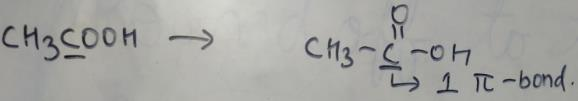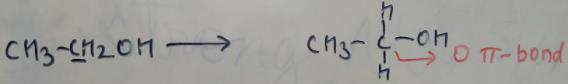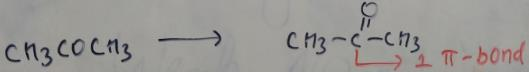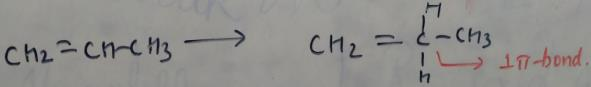From this figure, we can clearly see that in CH3CH2OH, we have no pi bond. While other compounds have pi bond. For carbon, only sp3 hybridization has no pi bond. So CH3CH2OH has sp3 hybridized carbon atom.

QUESTION: 2

### Which of the following is paramagnetic?  [NEET 2013]

Solution:

Paramagnetic species are those who have an unpaired electron. For CO, we have 14 electrons. For O2- we have 17 electrons. For CN- we have 14 electrons and for NO+ we have 14 electrons. So, we can see that only O2- have an unpaired electron.

QUESTION: 3

### A square planar complex is formed by hybridisation of which atomic orbital?  [AIEEE-2002]

Solution:

For square planar shape, we need all the orbitals lyng in a single plane. In option b s, px, py and dx2-y2 all lie in a single plane. This will give us a square plane shape.

QUESTION: 4

The reason for double helical structure of DNA is operation of:

[AIEEE-2003]

Solution:

Due to H-bonding, DNA structures itself in a double helix. The two polynucleotide chains or strands of DNA are linked up by hydrogen bonding between the nitrogenous base molecules of their nucleotide monomers.

QUESTION: 5

Which one of the following pairs of molecules will have permanent dipole moments for both members ?

Solution:

In the given set, NO2 and O3 are non-linear. O3 and NO2 both are bent shaped molecules. So, they will have permanent dipole moments.  CO2 is a linear molecule with sp hybridisation. So it won't have any dipole moment. SiF4 has tetrahedral shape but all bonds are identical and so that cancel out each other resulting in net dipole moment - 0

QUESTION: 6

The pair of species having identical shapes for molecules of both species is:

[AIEEE-2003]

Solution:

Both XeF2 & CO2 have linear structures. Both are actually linear Tri-atomic molecules.

QUESTION: 7

The correct order of bond angles (smallest first) in H2S, NH3, BF3 and SiH4 is:

[AIEEE-2004]

Solution:

For H2S, bond angle is around 90°. SiH4 is sp3 hybridized with no lone pair, so the bond angle is 109.28’. In NH3, due to the presence of lone pair on central N atom, bond angle decreases from 109°28’. BF3 is sp2 hybridized, so its bond angle is 120.So the order is;-
BF3 > SiH4 > NH3 > H2S
120   109     107     92.5

QUESTION: 8

The bond order in NO is 2.5 while that in NO+ is 3. Which of the following statements is true for these two species?

[AIEEE-2004]

Solution:

Bond length is inversely proportional to bond -order bond order in NO+  = 3, NO = 2.5Thus bond length in NO> NO+.Bond length is inversely proportional to bond -order bond order in NO+  = 3, NO = 2.5Thus bond length in NO> NO+

QUESTION: 9

The states of hybridization of boron and oxygen atoms in boric acid (H3BO3) are respectively:

[AIEEE-2004]

Solution:

According to me the correct option is b and not say because in boric acid Boron is sp2 hybridised due to an filled orbital while oxygen is sp3 hybridised.

QUESTION: 10

Which one of the following has the regular tetrahedral structure?

[AIEEE-2004]

Solution: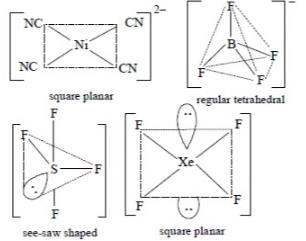From the above figure, we can see that BF4- has regular tetrahedral structure.

QUESTION: 11

The maximum number of 90° angles between bond pair-bond pair of electrons is observed in:

[AIEEE-2004]

Solution: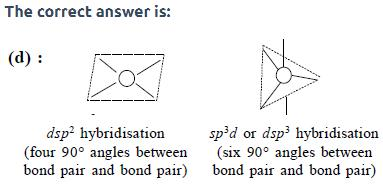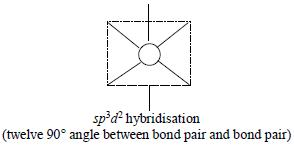QUESTION: 12

Beryllium and aluminium exhibit many properties which are similar. But, the two elements differ in:

[AIEEE-2004]

Solution:

Be (Z = 4) has maximum covalency of 4 while Al (Z = 13) has maximum covalency of 6.

QUESTION: 13

Lattice energy of an ionic compound depends upon:

[AIEEE-2005]

Solution:

Lattice energy of a compound depends on both charge and size with charge the first priority.

QUESTION: 14

The molecular shapes of SF4, CF4 and XeF4 are:

[AIEEE-2005]

Solution:

Hybridisation of all compounds
Hybridisation = valence electrons +sigma bonds/2
for SF4 = 6+4/2 =5 which means sp3d hybridisation , Now to get lone pairs subtract bonds from this number which is 5-4 =1 that's why 1 lone pair on SF4
for CF4 = 4+4/2 = 4, sp3 hybridisation
lone pairs = 4-4 =0
for XeF4 = 8+4/2 = 6, sp3d2 hybridisation
lone pairs = 6-4 =2
SF4 has sea-saw shape where one lone pair is in triangular plane.
CF4 is tetrahedral in shape because of sp3 hybridisation
XeF4 has square planar structure and 2 lone pairs are up & down of square plane.

QUESTION: 15

Of the following sets which one does not contain isoelectronic species?

[AIEEE-2005]

Solution:

In option a, b and d all  species have the same no of electrons.(There are 50 electrons in option a, 14 electrons in option b and 32 electrons in option d)However, SO3-2 has 42 electrons while CO3-2 and NO3- have 32 electrons. SO option c doesn’t contain isoelectronic species.

QUESTION: 16

The number and type of bond between two carbon atom in calcium carbide are:

[AIEEE-2005]

Solution: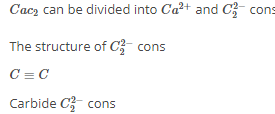There is a triple bond , between two carbons

Among three bonds one one is sigma, bond and the remaining two are π bond.

QUESTION: 17

Which of the following molecules/ions does not contain unpaired electron ?

[AIEEE-2006]

Solution:

O2 has two unpaired electron in the doubly degenerate 1πg* orbital. In O22-, the two electrons are accommodated in the 1ng* orbital meaning the orbitals are full and hence all the electrons are paired. So no unpaired electrons are present.

QUESTION: 18

Among the following mixtures, dipole-dipole as the major interaction, is present in:

[AIEEE-2006]

Solution:

Acetonitrile and Acetone both are polar molecules hence dipole dipole interaction exist between them between KCL and water Ion dipole interaction is found and in Benzene ethanol and benzene carbon tetrachloride dispersion force is present.

QUESTION: 19

A metal, M foms chlorides in its +2 and +4 oxidation states. Which of the following statements about these chlorides is correct?

[AIEEE-2006]

Solution:

Because according to Fajan's rule, compounds of metal in lower oxidation state are more ionic than those in a higher state.

QUESTION: 20

In which of the following molecules/ions are all the bonds not equal?

[AIEEE-2006]

Solution:

Out of the four, SF4 will not have equal bonds, because of it structure .Trigonal bipyramidal. Sulfur in SF4 is in the formal +4 oxidation state. sulfur has total of six valence electrons, two form a lone pair. The fluorine-sulfur-fluorine bond angles are 120° in the trigonal plane and 90° to the two fluorine atoms above and below the plane.

QUESTION: 21

The decreasing value of bond angles from NH3 (106)° to SbH3 (101)° down group-15 of the periodic table is due to:

[AIEEE-2006]

Solution:

With the increase in electronegativity, bond pairs move farther and farther away from the central atom. As a result bp-bp repulsion decreases and the angle decreases accordingly.

QUESTION: 22

In which of the following ionizion processes, the bond order has increased and the magnetic behaviour has changed

[AIEEE-2007]

Solution:

N2: bond order 3, paramagnetic
N2-: bond order 2.5, paramagnetic
C2: bond order 2, diamagnetic
C2+: bond order 1.5, paramagnetic
NO: bond order 2.5, paramagnetic
NO+: bond order 3, paramagnetic
O2: bond order 2, paramagnetic
O2+: bond order 2.5, paramagnetic
Therefore, option a is correct

QUESTION: 23

Which of the following hydrogen bonds is the strongest

[AIEEE-2007]

Solution:

Since F is most electronegative,so H bon by its hydride will be most strong.

QUESTION: 24

The charge/size ratio of a cation determines its polarzing power. Which one of the following sequences represents the increasing order of the polarizing power of the cationic species, K+, Ca+2 Mg+2, Be+2

[AIEEE-2007]

Solution:

Since, Be belongs to the 2nd group, so its size is the least. Therefore its polarisability power will be most. Mg is in 3rd period and its polarisability power will be less than Be. FO rCA and K, both belong to the same period but Ca has more charge. SO its polarisability will be more than k .

QUESTION: 25

Give reason carbon oxygen bond lengths in formic acid are 1.23 Å & 1.36Å and both the carbon oxygen bonds in sodium formate have the same value i.e. 1.27 Å                [JEE' 88]

Solution:

Carbon-oxygen bond lengths in formic acid are 1.23 Å and 1.36 Å, but both the carbon-oxygen bonds in sodium formate have the same value, 1.27 Å. In formic acid the carbon-oxygen bond lengths are different due to hydrogen bonding.

QUESTION: 26

In allene (C3H4), the type(s) of hybridisation of the carbon atoms is (are)

Solution:

The structure of Allene is H2C=C=CH2. We can see that there is a sp hybridised carbon atom in the middle and 2 sp2 hybridised carbon atom on extreme sides. So the correct answer is sp and sp2

QUESTION: 27

Mark True or False: BeCl2 can be easily hydrolyed               [JEE'99]

Solution:

BeCl2 is hydrolysed due to high polarizing power and presence of vacant p-orbitals in Be-atom Chromic acid. Therefore CrO3 is acid anhydride of H2Cr2O4.

QUESTION: 28

o-hydroxybenzaldehyde is a liquid at room temperature, while p-hydroxybenzaldehyde is a high melting solid due to           [JEE' 99]

Solution:

o-hydroxybenzadehyde is a liquid at room temperature while p-hydroxybenzaldehyde is high melting solid because there is intramolecular H-bonding in o-hydroxybenzaldehyde (weak) while intermolecular H-bonding in p-isomer (strong).

QUESTION: 29

N2, O2, F2, Cl2 in increasing order of bond dissociation energy.                   [JEE' 88]

Solution:

Bond dissociation energy of F2 is less than Cl2
F2 < Cl2
Bond dissociation energies of O2 (O=O) and N2(N=N) are more than Cl2 or F2 which contains only single bonds. Out of N2 (with a triple bond) and O2 (with a double bond), bond dissociation energy of N2 is more than O2. Therefore correct order is : F2<Cl2<O2<N2

QUESTION: 30

Arrange in increasing order of dipole moment. [JEE'96]

(I) Toluene, (II) m-dichcorobenzene (III) O-dichlorobenzene (IV) P-dichlorobenzene

Solution:

The increasing order of dipole moment is IV<I<II<III.

o−, m−, p− derivates has α=60o, 120o and 180o and thus, resultant vector has zero dipole moment in p-derivative because of symmetrical structure. Also dipole moment of m-dichlorobenzene is more than toluene. o− and m−dichlorobenzene have higher dipole moments than toluene due to high electronegativity of chlorine than CH3​ group. Further, to the o-dichlorobenzene has higher dipole moment due to lower bond angle than the m-isomer.